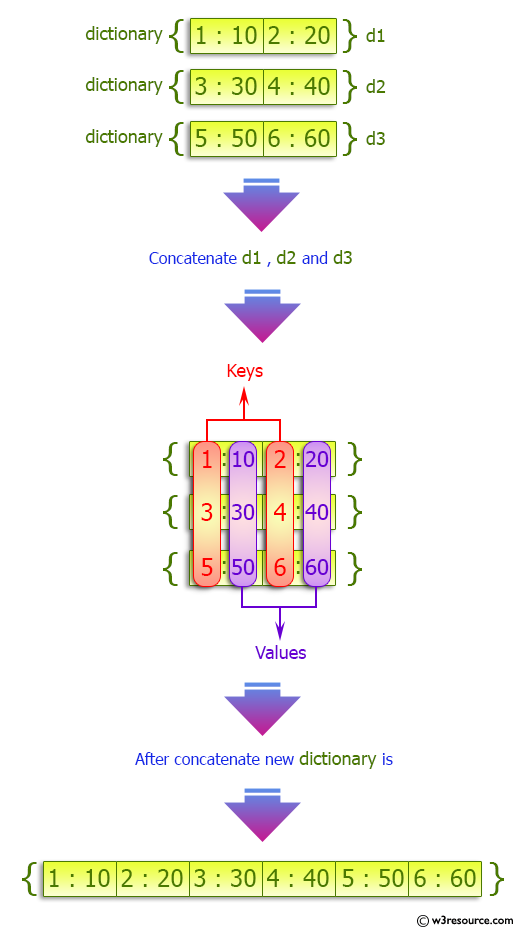﻿ Python: Concatenate following dictionaries to create a new one - w3resource# Python: Concatenate following dictionaries to create a new one

## Python dictionary: Exercise-3 with Solution

Write a Python program to concatenate following dictionaries to create a new one.

Sample Solution:-

Python Code:

``````dic1={1:10, 2:20}
dic2={3:30, 4:40}
dic3={5:50,6:60}
dic4 = {}
for d in (dic1, dic2, dic3): dic4.update(d)
print(dic4)
```
```

Sample Output:

```{1: 10, 2: 20, 3: 30, 4: 40, 5: 50, 6: 60}
```

Pictorial Presentation:## Visualize Python code execution:

The following tool visualize what the computer is doing step-by-step as it executes the said program:

Python Code Editor:

Have another way to solve this solution? Contribute your code (and comments) through Disqus.

What is the difficulty level of this exercise?

Test your Programming skills with w3resource's quiz.

﻿

## Python: Tips of the Day

Decapitalizes the first letter of a string:

Example:

```def tips_decapitalize(s, upper_rest=False):
return s[:1].lower() + (s[1:].upper() if upper_rest else s[1:])
print(tips_decapitalize('PythonTips'))
print(tips_decapitalize('PythonTips', True))
```

Output:

```pythonTips
pYTHONTIPS
```